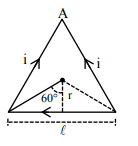# A current of 1.5 A is flowing through a triangle,Question:

A current of $1.5 \mathrm{~A}$ is flowing through a triangle, of side $9 \mathrm{~cm}$ each. The magnetic field at the centroid of the triangle is:

(Assume that the current is flowing in the clockwise direction.)

1. $3 \times 10^{-7} \mathrm{~T}$, outside the plane of triangle

2. $2 \sqrt{3} \times 10^{-7} \mathrm{~T}$, outside the plane of triangle

3. $2 \sqrt{3} \times 10^{-5} \mathrm{~T}$, inside the plane of triangle

4. $3 \times 10^{-5} \mathrm{~T}$, inside the plane of triangle

Correct Option: , 4

Solution:$\mathrm{B}=3\left[\frac{\mu_{0} \mathrm{i}}{4 \pi \mathrm{r}}\left(\sin 60^{\circ}+\sin 60^{\circ}\right)\right]$

$\tan 60^{\circ}=\frac{\ell / 2}{r}$

Where $\mathrm{r}=\frac{9 \times 10^{-2}}{2 \sqrt{3}} \mathrm{M}$

$\therefore B=3 \times 10^{-5} \mathrm{~T}$

Current is flowing in clockwise direction so, $\overrightarrow{\mathrm{B}}$ is inside plane of triangle by right hand rule.